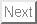Proceeding of International Conference
Tetsushi Ueta, Quentin Brandon, Akihisa Tamura, Takuji Kousaka, Bifurcation and chaos in switched autonomous systems, Proc. NDES'08, 25-28, Nizhny Novgorod, July 2008.
Abstract: In the fields of circuits and systems, there are many discontinuous situations in their characteristics or operations. Rather we affirmatively utilize these property to obtain quick responses or apply various signal processing. Switching, impulsive trains, break points, bending points, borders, hystereses, threshold values, and cut-in voltages are example factors making the systems discontinuous. Especially analysis on a nonlinear circuit with these discontinuity becomes a very difficult problem. In addition, continuous characteristics for derivatives of the state and parameters are required for the general way of analysis for nonlinear systems, thus even the case using a periodic rectangular wave source cannot be treated directly. Bifurcation problems in circuits with above characteristics are discussed within very limited categories1;2. Piecewise linear systems have been intensively investigated for two decades because solutions the systems and their derivatives are obtained exactly, for instance, various converters, Chua's circuit3, hysteresis circuits and so on. Only a numerical computation of the return time or the period is required for bifurcation analysis. Approximation of the piecewise linear characteristics by smooth functions is often tried, especially for the Chua's circuit. While, discontinuous characteristics are also observed in physical systems, e.g., impulsive behavior in neuronal and biological activities. The problem is loosing continuity of the state, or its variations. In general, smooth functions are naturally assumed in analyzing process, in fact, without this assumption, it is unable to obtain derivatives of the state and its variations needed for Jacobian matrix in the shooting method. Above approximation is avoidance of this difficulty. Even if the given system equation and the state are continuous, computation of information about dynamical behavior requires continuity of their derivatives. Assume that we have now a piecewise autonomous nonlinear system. The system equation may be given in nonlinear ordinary differential equations with some conditional statements. Then the following equation is held piecewisely, such as dx/dt = fk(x), k = 0, 1, 2, . . .m, x(0) = x0. We have developed a general framework of bifurcation analysis for dynamical systems including discontinuous characteristics4. For piecewise nonlinear autonomous systems, more careful treatment is needed. Indeed the characteristic equation directly induced from the Jacobian matrix of the composite Poincar´e map does not contains correct multipliers of the limit cycle.